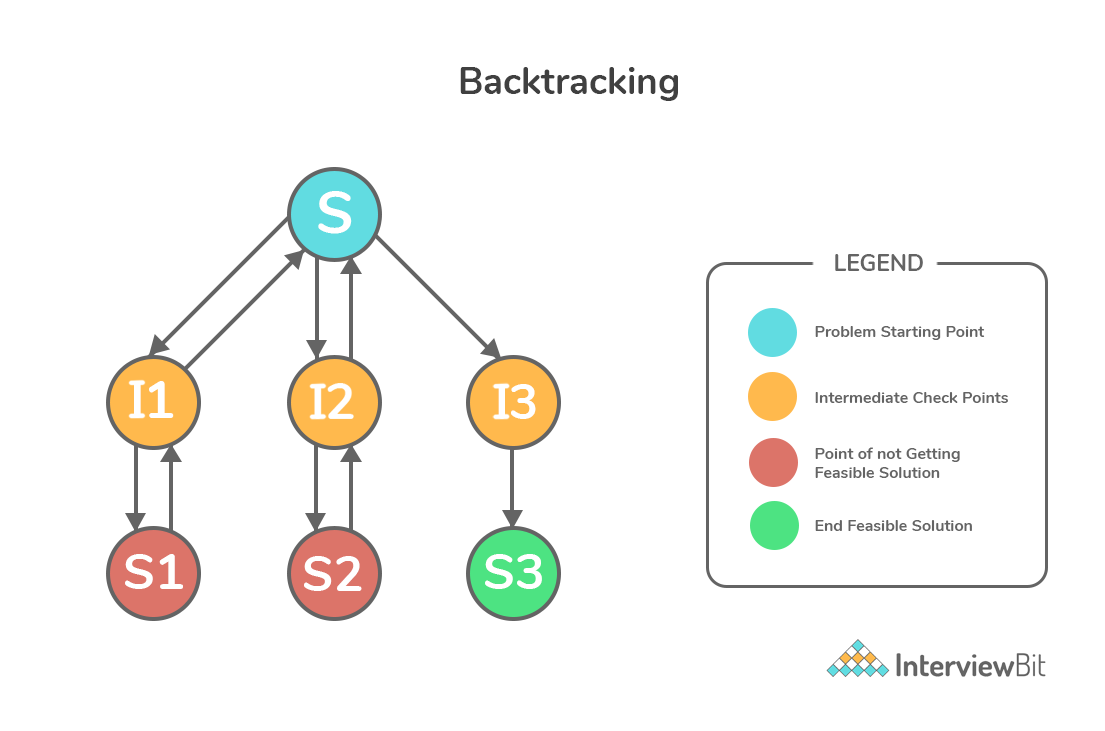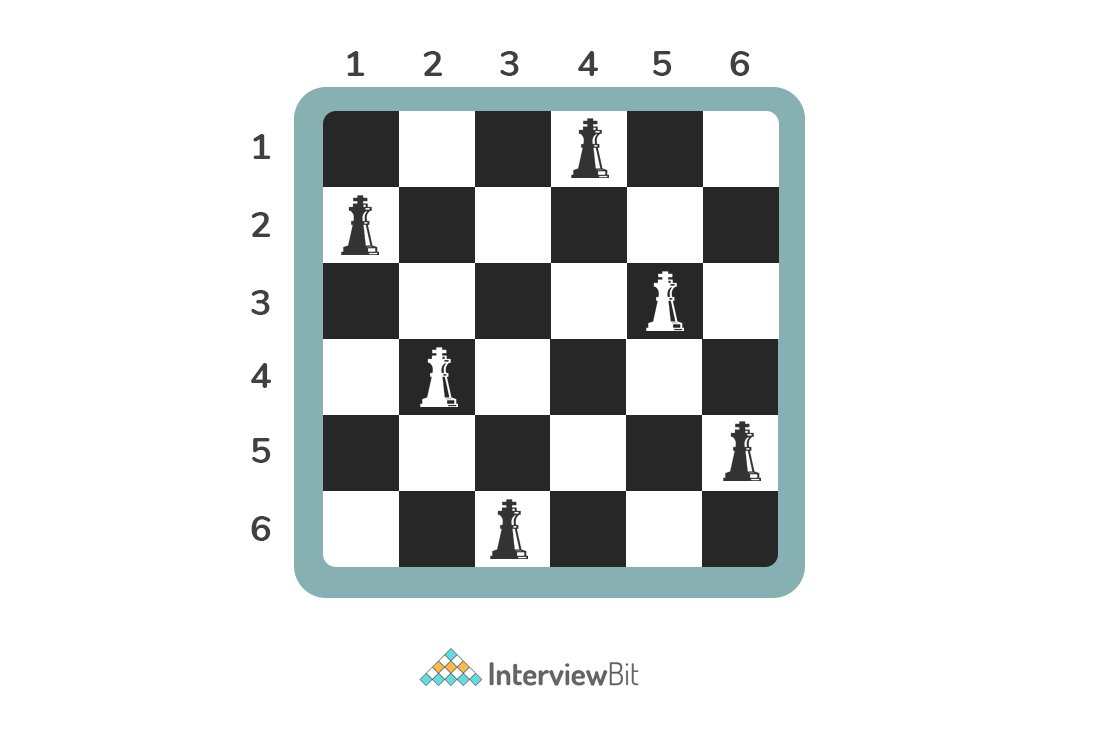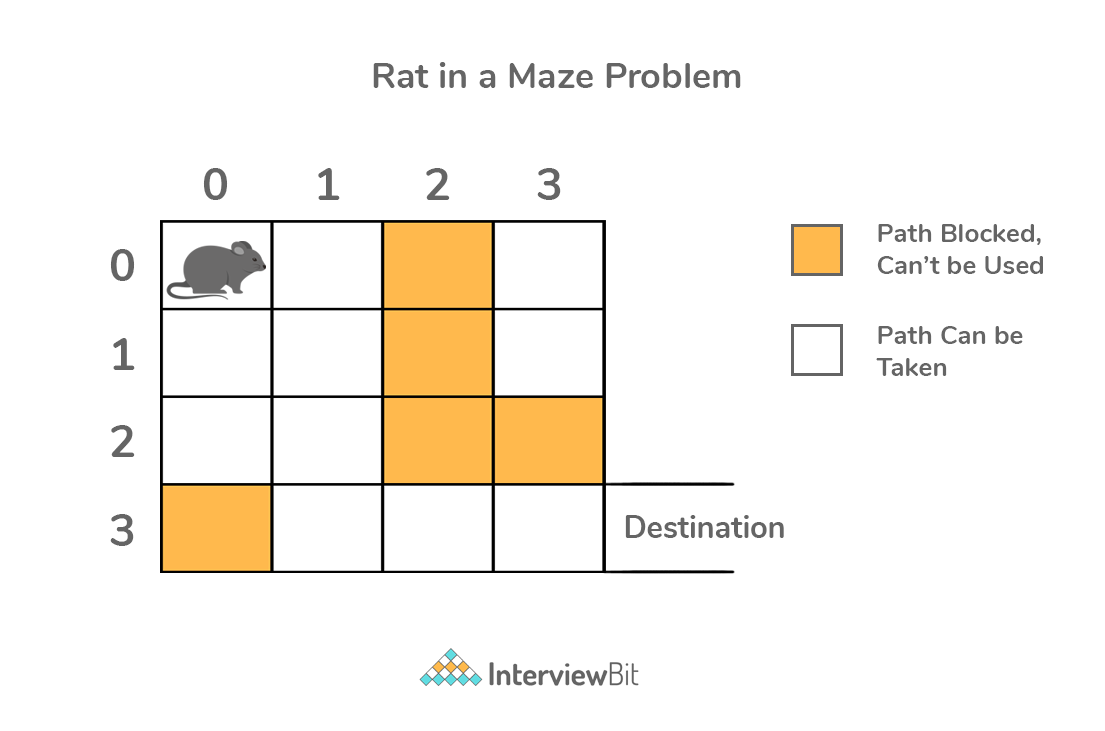## Welcome to Interviewbit, help us create the best experience for you!

Currently, You are a:College/University *
Enter the name of your college
Branch *
Year of completion *College/University *
Enter the name of your college
Branch *
Year of completion *Current Company *
Enter company name
Experience *## You're all set!Full name *
Email *

By creating an account, I acknowledge that I have read and agree to InterviewBit’s Terms and Privacy Policy .## Welcome back!

Email *# Backtracking

### What is Backtracking?

• Backtracking is an algorithmic technique that considers searching in every possible combination for solving a computational problem.
• It is known for solving problems recursively one step at a time and removing those solutions that that do not satisfy the problem constraints at any point of time.
• It is a refined brute force approach that tries out all the possible solutions and chooses the best possible ones out of them.
• The backtracking approach is generally used in the cases where there are possibilities of multiple solutions.
``The term backtracking implies - if the current solution is not suitable, then eliminate that and backtrack (go back) and check for other solutions.``

### Backtracking - How it works?

In any backtracking problems, the algorithm tries to find a path to the feasible solution which has some intermediary checkpoints. In case they don’t lead to the feasible solution, the problem can backtrack from the checkpoints and take another path in search of the solution. Consider the below example:• Here S is the starting point of the problem. We start from S, we go to find solution S1 via the intermediate point I1. But we find that the solution S1 is not a feasible solution to our problem. Hence, we backtrack (go back) from S1, go back to I1, go back to S and then check for the feasible solution S2. This process happens till we arrive at a feasible solution.
• Here, S1 and S2 are not the feasible solutions. Only S3 is a feasible solution as per our example. When we look at this example, we can see that we traverse through all possible combinations, till we arrive at the feasible solution. This is why, we say that backtracking is a brute-force algorithmic technique.
• The above tree representation of a problem is called as a “space state tree”. It represents all possible states (solution or non-solution) of that given problem.
• The final algorithm can be summarised as:
Step 1 − if current point is a feasible solution, return success
Step 2 − else if all paths are exhausted (i.e current point is an end point), return failure, since we have no feasible solution.
Step 3 − else if current point is not an end point, backtrack and explore other points and repeat above steps.

### Important tutorials

1. Recursion Basics Using Factorial
2. Complexity Analysis Of Recursive Programs
3. Why Recursion Is Not Always Good
4. Time Complexity Analysis Of Recursion
5. Space Complexity Analysis Of Recursion
6. Maze Traversal Algorithm Using Backtracking
7. Graph Coloring Algorithm Using Backtracking

## Serious about Learning Programming ?

Learn this and a lot more with Scaler Academy's industry vetted curriculum. Attend a Free Live Class today!
Examples

## Backtracking Problems

Maths and backtracking
Problem Score Companies Time Status
Maximal String 200
57:51
Kth Permutation Sequence 350 76:22
Gray Code 350
58:10
Subsets
Problem Score Companies Time Status
Subset 250 61:56
Combination Sum 300 62:33
Combination Sum II 300 41:41
Combinations 300 46:07
Subsets II 300 41:00
Bruteforce builder
Problem Score Companies Time Status
Letter Phone 250 53:36
Palindrome Partitioning 300 67:57
Generate all Parentheses II 350
52:28
Permutations
Problem Score Companies Time Status
Permutations 350 39:38
Game solving
Problem Score Companies Time Status
NQueens 550 74:27
Sudoku 700 69:19

Problem Score Companies Time Status
All Possible Combinations 300 26:55

### Types of Backtracking Problems:

Problems associated with backtracking can be categorized into 3 categories. They are:

• Decision Problems – Here, we search for a feasible solution.
• Optimization Problems – For this type, we search for the best solution.
• Enumeration Problems – We find set of all possible feasible solutions to the problems of this type.

### Backtracking Problem Identification

• Every problem that has clear and well established constraints on any objective solution which incrementally aids candidate to the solution and abandons a candidate (“backtracks”) whenever it determines that the candidate is not able to reach a feasible solution. Such problems can be solved by Backtracking. The backtracking algorithms are generally exponential in nature with regards to both time and space.
• However, most of the commonly discussed problems, can be solved using other popular algorithms like Dynamic Programming or Greedy Algorithms in O(n), O(logn) or O(n* logn) time complexities in order of input size. Hence, in such cases, usage of backtracking becomes an overkill.
• But, there still remain some problems that only have backtracking algorithms as the means of solving them till date.
• Consider a real life scenario. We have three boxes and we are told that only one of them has a gold coin in it and we do not know exactly which box has it. So, in order to identify which box has the coin, we will have no other option than opening all the boxes one by one.
•• The first box is checked first. If it does not contain the coin, we close it and check the second box and so on until we find the coin. This is nothing but utilisation of backtracking algorithm in real life. It is the process of solving all sub-problems one by one to reach the best possible solution for any given problem.
• Let us take a technical example and understand backtracking more clearly. Given an instance of any problem `P` and data `D` corresponding to the instance, all the constraints that are to be satisfied for solving the problem as `C`. The backtracking algorithm will then be:
• The algorithm begins to build up a solution, starting with an empty solution set `S`. `S = {}`
• All possible moves are added to S one by one. Firstly, we add to `S` the first move that is left. This now creates a new sub-tree `s` in the state space tree.
• Check if `S+s` is valid by seeing if it satisfies each of the constraints defined in `C`.
• If `S+s` is valid, then the sub-tree `s` is “eligible” to add more “children”.
• Else, the entire sub-tree `s` is useless, so go back to step 1 using argument `S`.
• In case of “eligibility” of the newly formed sub-tree `s`, go back to step 1 using argument `S+s`.
• If `S+s` returns that it is a solution for the entire data `D`. Return the result and terminate the algorithm.
• If not, then return that there doesn’t exist any possible solution for the current `s` and hence discard it.

### Application

Backtracking has found numerous applications for solving real life commonly encountered problems by satisfying certain constraints. Problems like crosswords, verbal arithmetic, Sudoku, and many other puzzles can be solved by using backtracking approach.

• N-Queens Problem: Backtracking is also used in solving N queens problem in N*N chessboard. The problem basically deals with placing N queens on NN board without threatening each other. That is no two queens share the same row, column, or diagonal on the chessboard.• By using backtracking, the idea for solving this problem is to place queens 1 by 1 in different columns, starting from the leftmost column. While placing the queen, we check whether it is safe to place the queen at that cell by checking with already placed queens. If we find a row for which there are no queens placed in the current column under consideration, we mark this cell (ith row and jth column) as part of the solution. If we are not able to find any such rows, then we backtrack and return false.
• Maze Problems:: Backtracking is used to solve maze related problems. The most common problem is rat in a maze problem. The problem statement says that a maze in the form of N*N binary matrix of blocks is given where source block (starting point) is the top left most block i.e., maze and destination block (ending point) is bottom rightmost block i.e., maze[N-1][N-1]. A rat begins to move from start point and has to reach the destination but it can move only in 2 directions - forward and down. Additionally, the maze matrix has certain dead ends defined. The value 0 means the cell is a dead-end and 1 means the cell can be used as path to move from source to destination.• Graph coloring problem: Read More
• Backtracking is also used in graphs to find Hamiltonian cycles. A Hamiltonian cycle (or Hamiltonian circuit) is a Hamiltonian Path (path which visits each vertex exactly once) in such a way that there exists an edge (in the graph) from the last vertex to the first vertex of the Hamiltonian Path. This has a lot of application in the field of computer graphics.
• Apart from these, backtracking is also used in the area of solving cryptarithmetic puzzles, magnet puzzles, finding subset sum problems and many more!

### FAQ

• What are backtracking algorithms?
• Backtracking is an algorithmic technique for finding all solutions to some computational problems that have certain constraints and incrementally builds candidates to the solutions while abandoning a candidate if it does not lead to valid solutions.
• How Backtracking algorithms work?
• Backtracking uses recursive approach to find feasible solution by building a solution incrementally with time and removing the solutions that dont lead to feasible solution for the problem based on the constraints given.
• When is backtracking used?
• Backtracking is normally used when we are faced with a multiple number of options and we have to choose one among them based on the constraints given. After the choice we will be having a new set of options which is where the recursion comes to the rescue. The procedure is repeated untill we get a feasible solution.
• Is backtracking better than brute force algorithms?
• Brute force algorithms are those which computes every possible solution to a problem and then selects the best one among them that fulfills the given requirements.
• Whereas, backtracking is a refined brute force technique where the implicit constraints are evaluated after every choice (not as in brute force where evaluation is done after all solutions have been generated). This means that potential non-satisfying solutions can be rejected before the computations have been ‘completed’.
• What is the time complexity of backtracking algorithm?
• The time complexity of the algorithm will be a measure specific to what problem we are trying to solve.
• What is the difference between recursion and backtracking?
• In recursion, a function simply calls itself until reaches a base case.
• Whereas, in backtracking we use recursion for exploring all the possibilities until we get the best and feasible result for any given problem.